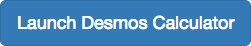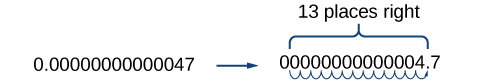## Scientific Notation

### Learning Outcomes

• Convert standard notation to scientific notation.
• Convert from scientific to standard notation.
• Apply scientific notation in an application.Recall at the beginning of the section that we found the number $1.3\times {10}^{13}$ when describing bits of information in digital images. Other extreme numbers include the width of a human hair, which is about 0.00005 m, and the radius of an electron, which is about 0.00000000000047 m. How can we effectively work read, compare, and calculate with numbers such as these?

A shorthand method of writing very small and very large numbers is called scientific notation, in which we express numbers in terms of exponents of 10. To write a number in scientific notation, move the decimal point to the right of the first digit in the number. Write the digits as a decimal number between 1 and 10. Count the number of places n that you moved the decimal point. Multiply the decimal number by 10 raised to a power of n. If you moved the decimal left as in a very large number, $n$ is positive. If you moved the decimal right as in a small large number, $n$ is negative.

For example, consider the number 2,780,418. Move the decimal left until it is to the right of the first nonzero digit, which is 2.We obtain 2.780418 by moving the decimal point 6 places to the left. Therefore, the exponent of 10 is 6, and it is positive because we moved the decimal point to the left. This is what we should expect for a large number.

$2.780418\times {10}^{6}$

Working with small numbers is similar. Take, for example, the radius of an electron, 0.00000000000047 m. Perform the same series of steps as above, except move the decimal point to the right.Be careful not to include the leading 0 in your count. We move the decimal point 13 places to the right, so the exponent of 10 is 13. The exponent is negative because we moved the decimal point to the right. This is what we should expect for a small number.

$4.7\times {10}^{-13}$

### A General Note: Scientific Notation

A number is written in scientific notation if it is written in the form $a\times {10}^{n}$, where $1\le |a|<10$ and $n$ is an integer.

### Example: Converting Standard Notation to Scientific Notation

Write each number in scientific notation.

1. Distance to Andromeda Galaxy from Earth: 24,000,000,000,000,000,000,000 m
2. Diameter of Andromeda Galaxy: 1,300,000,000,000,000,000,000 m
3. Number of stars in Andromeda Galaxy: 1,000,000,000,000
4. Diameter of electron: 0.00000000000094 m
5. Probability of being struck by lightning in any single year: 0.00000143

### Try It

Write each number in scientific notation.

1. U.S. national debt per taxpayer (April 2014): $152,000 2. World population (April 2014): 7,158,000,000 3. World gross national income (April 2014):$85,500,000,000,000
4. Time for light to travel 1 m: 0.00000000334 s
5. Probability of winning lottery (match 6 of 49 possible numbers): 0.0000000715

## Converting from Scientific to Standard Notation

To convert a number in scientific notation to standard notation, simply reverse the process. Move the decimal $n$ places to the right if $n$ is positive or $n$ places to the left if $n$ is negative and add zeros as needed. Remember, if $n$ is positive, the value of the number is greater than 1, and if $n$ is negative, the value of the number is less than one.

### Example: Converting Scientific Notation to Standard Notation

Convert each number in scientific notation to standard notation.

1. $3.547\times {10}^{14}$
2. $-2\times {10}^{6}$
3. $7.91\times {10}^{-7}$
4. $-8.05\times {10}^{-12}$

### Try It

Convert each number in scientific notation to standard notation.

1. $7.03\times {10}^{5}$
2. $-8.16\times {10}^{11}$
3. $-3.9\times {10}^{-13}$
4. $8\times {10}^{-6}$

## Using Scientific Notation in Applications

Scientific notation, used with the rules of exponents, makes calculating with large or small numbers much easier than doing so using standard notation. For example, suppose we are asked to calculate the number of atoms in 1 L of water. Each water molecule contains 3 atoms (2 hydrogen and 1 oxygen). The average drop of water contains around $1.32\times {10}^{21}$ molecules of water and 1 L of water holds about $1.22\times {10}^{4}$ average drops. Therefore, there are approximately $3\cdot \left(1.32\times {10}^{21}\right)\cdot \left(1.22\times {10}^{4}\right)\approx 4.83\times {10}^{25}$ atoms in 1 L of water. We simply multiply the decimal terms and add the exponents. Imagine having to perform the calculation without using scientific notation!

When performing calculations with scientific notation, be sure to write the answer in proper scientific notation. For example, consider the product $\left(7\times {10}^{4}\right)\cdot \left(5\times {10}^{6}\right)=35\times {10}^{10}$. The answer is not in proper scientific notation because 35 is greater than 10. Consider 35 as $3.5\times 10$. That adds a ten to the exponent of the answer.

$\left(35\right)\times {10}^{10}=\left(3.5\times 10\right)\times {10}^{10}=3.5\times \left(10\times {10}^{10}\right)=3.5\times {10}^{11}$

### Example: Using Scientific Notation

Perform the operations and write the answer in scientific notation.

1. $\left(8.14\times {10}^{-7}\right)\left(6.5\times {10}^{10}\right)$
2. $\left(4\times {10}^{5}\right)\div \left(-1.52\times {10}^{9}\right)$
3. $\left(2.7\times {10}^{5}\right)\left(6.04\times {10}^{13}\right)$
4. $\left(1.2\times {10}^{8}\right)\div \left(9.6\times {10}^{5}\right)$
5. $\left(3.33\times {10}^{4}\right)\left(-1.05\times {10}^{7}\right)\left(5.62\times {10}^{5}\right)$

Watch the following video to see more examples of writing numbers in scientific notation.

### Try It

Perform the operations and write the answer in scientific notation.

1. $\left(-7.5\times {10}^{8}\right)\left(1.13\times {10}^{-2}\right)$
2. $\left(1.24\times {10}^{11}\right)\div \left(1.55\times {10}^{18}\right)$
3. $\left(3.72\times {10}^{9}\right)\left(8\times {10}^{3}\right)$
4. $\left(9.933\times {10}^{23}\right)\div \left(-2.31\times {10}^{17}\right)$
5. $\left(-6.04\times {10}^{9}\right)\left(7.3\times {10}^{2}\right)\left(-2.81\times {10}^{2}\right)$

### Example: Applying Scientific Notation to Solve Problems

In April 2014, the population of the United States was about 308,000,000 people. The national debt was about \$17,547,000,000,000. Write each number in scientific notation, rounding figures to two decimal places, and find the amount of the debt per U.S. citizen. Write the answer in both scientific and standard notations.

### Try It

An average human body contains around 30,000,000,000,000 red blood cells. Each cell measures approximately 0.000008 m long. Write each number in scientific notation and find the total length if the cells were laid end-to-end. Write the answer in both scientific and standard notations.

## Contribute!

Did you have an idea for improving this content? We’d love your input.